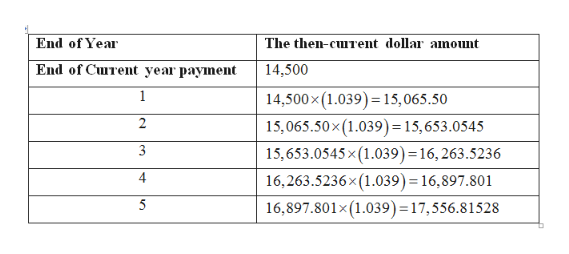# Your department is budgeting miscellaneous expenses for the next 5 years. Your best guess at the annual inflationrate is 3.9 percent, and the combined MARR is 15 percent. Expenses currently run \$14,500 per year. Assume thatexpenses are end-of-year payments.a. Determine the then-current dollar amounts for years 1, 2, 3, 4, and 5.b. Determine the constant dollar amount for years 1, 2, 3, 4, and 5.Year 2005 2006 2007 2008 2009Rate % 18.853% 15.995% 10.665% "16.220% "12.723%574 CHAPTER 12 / INFLATION EFFECTSc. Determine the PW of the then-current dollar amounts.d. Determine the PW of the constant dollar amounts.

Question
23 views

Your department is budgeting miscellaneous expenses for the next 5 years. Your best guess at the annual inflation
rate is 3.9 percent, and the combined MARR is 15 percent. Expenses currently run \$14,500 per year. Assume that
expenses are end-of-year payments.
a. Determine the then-current dollar amounts for years 1, 2, 3, 4, and 5.
b. Determine the constant dollar amount for years 1, 2, 3, 4, and 5.
Year 2005 2006 2007 2008 2009
Rate % 18.853% 15.995% 10.665% "16.220% "12.723%
574 CHAPTER 12 / INFLATION EFFECTS
c. Determine the PW of the then-current dollar amounts.
d. Determine the PW of the constant dollar amounts.

check_circle

Step 1

a.

The expenses of the department are given to be \$14,500 at the current period and the rate if inflation is given to be 3.9 percent. The combined MARR is also given which is 15 percent. The expenses are made at the end of the year. Thus, the then current dollar amount of the year 1 can be calculated by multiplying the expenses that are running current with the 1+inflation rate which can be calculated as follows:

Step 2

Thus, the then current value of dollar for year 1 is \$15,065.50. The value for the year 2 can be calculated by the same method and using the then-current value of year 1 as the current expenses. This can be repeated by year after year to calculate the then-current dollar value for year 1 to 5 and the table can be completed as follows:help_outlineImage TranscriptioncloseThe then-curent dollar anoumt End of Year End of Curent year payment 14,500 14,500x (1.039)15,065.50 1 2 15,065.50x (1.039) = 15, 653.0545 3 15,653.0545 x (1.039) = 16, 263.5236 4 16,263.5236x(1.039) = 16,897.801 16,897.801x (1.039) = 17, 556.8 1528 fullscreen
Step 3

b.

The constant dollar amount for all the year will be the same as the inflation rate will not affect the constant dollar amount. Its only when the inflation rate affects the expenses, there will be chan...

### Want to see the full answer?

See Solution

#### Want to see this answer and more?

Solutions are written by subject experts who are available 24/7. Questions are typically answered within 1 hour.*

See Solution
*Response times may vary by subject and question.
Tagged in